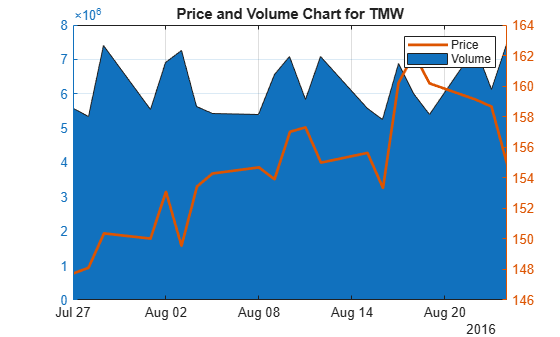# volarea

Price and volume chart

`volarea` is updated to accept data input as a matrix, `timetable`, or `table`.

The syntax for `volarea` has changed. Previously, when using table input, the first column of dates could be a datetime array, date character vectors, or serial date numbers, and you were required to have specific number of columns.

When using table input, the new syntax for `volarea` supports:

• No need for time information. If you want to pass in date information, use timetable input.

• No requirement of specific number of columns. However, you must provide valid column names. `volarea` must contain a column named `‘price’` (case insensitive).

## Syntax

``volarea(Data)``
``h = volarea(ax,Data)``

## Description

example

````volarea(Data)` plots a chart from a series of price and traded volume of a security.```

example

````h = volarea(ax,Data)` adds an optional argument for `ax`. ```

## Examples

collapse all

Load the file `SimulatedStock.mat`, which provides a timetable (`TMW`) for financial data for TMW stock. This example shows how to plot asset date, price, and volume on a single axis, given asset TMW containing asset price and volume in most recent 21 days. Note that the variable name of asset price is be renamed to `'Price'` (case insensitive).

```load SimulatedStock.mat; TMW.Properties.VariableNames{'Close'} = 'Price'; volarea(TMW(end-20:end,:)) title('Price and Volume Chart for TMW')```## Input Arguments

collapse all

Data for prices and traded volume, specified as a matrix, table, or timetable. For matrix input, `Data` is an `M`-by-`2` matrix of prices and traded volume. Timetables and tables with `M` rows must contain variables named `'Price'` and `'Volume'` (case insensitive).

Data Types: `double` | `table` | `timetable`

(Optional) Valid axis object, specified as an axes object. The volarea plot is created in the axes specified by `ax` instead of in the current axes (`ax = gca`). The option `ax` can precede any of the input argument combinations.

Data Types: `object`

## Output Arguments

collapse all

Graphic handle of the figure, returned as a handle object.

## Version History

Introduced in R2008a

expand all